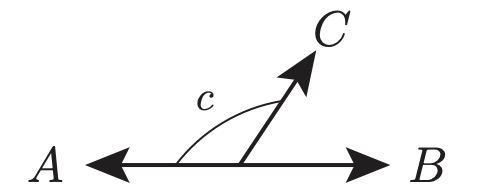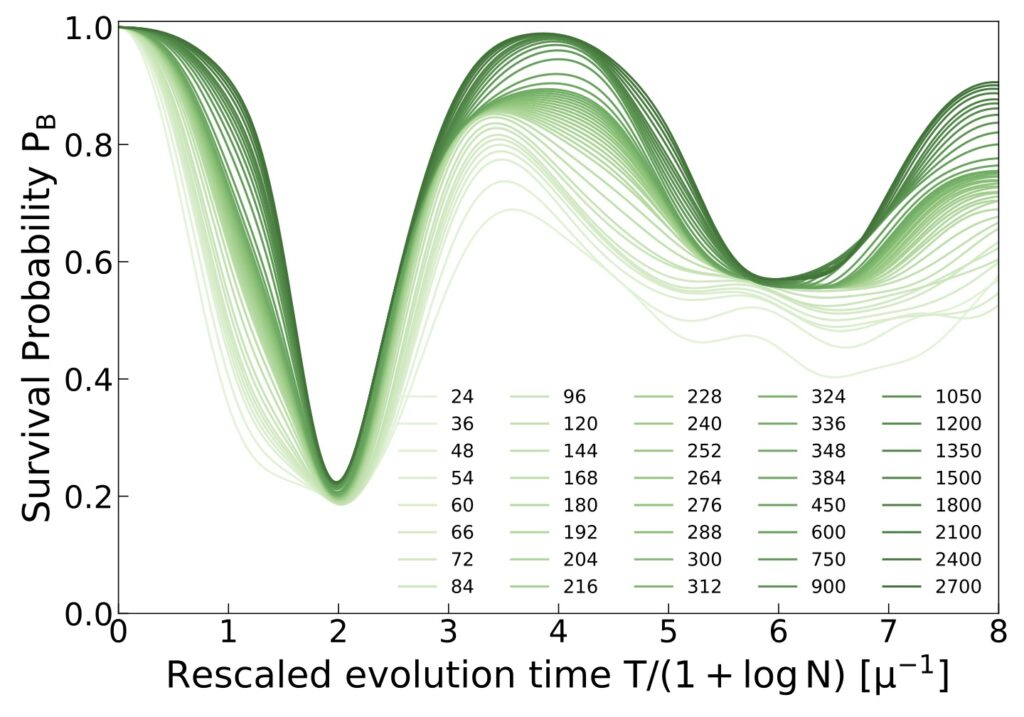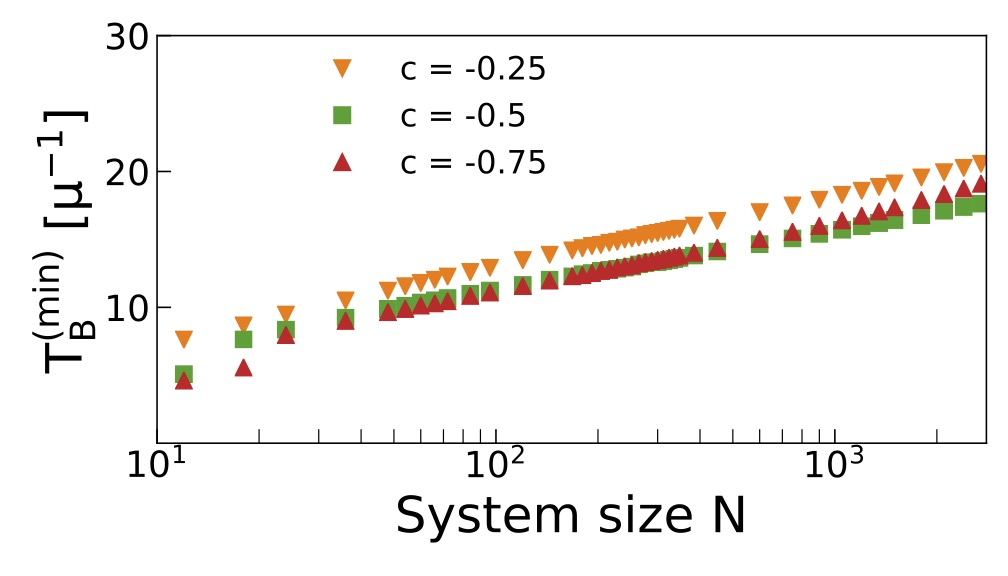# Entanglement and correlations in fast collective neutrino flavor oscillations

Prepared by Ermal Rrapaj

Collective neutrino oscillations play a crucial role in transporting lepton flavor in astrophysical settings like supernovae and neutron star binary merger remnants, which are characterized by large neutrino densities. In these settings, simulations in the mean-field approximation show that neutrino-neutrino interactions can overtake vacuum oscillations and give rise to fast collective flavor evolution on time-scales inversely proportional to the local neutrino number density. In this work, we study the full out-of-equilibrium flavor dynamics in simple multi-angle geometries displaying fast oscillations in the mean field linear stability analysis.Beams A and B are antiparallel, and beam C forms an angle with beam as shown in the figure.

Focusing on simple initial conditions, we analyze the production of pair correlations and entanglement in the complete many-body-dynamics as a function of the number of neutrinos in the system, for up to thousands of them. Similarly to simpler geometries with only two neutrino beams, we identify three regimes: stable configurations with vanishing flavor oscillations, marginally unstable configurations with evolution occurring on long time scales, proportional to the square root of number of particles, and unstable configurations showing flavor evolution on short time scales proportional to the logarithm of the number of particles.Time evolution of survival probability in beam B for the initial state in Setup II in the paper with cosine between beams A and C, c=-0.5. The plot uses a rescaled time variable (darker colors indicate larger values of N) to highlight the system size dependence.Time to reach the first minimum in the survival probability in beam B for the initial state in Setup II and different angular distributions as function of system size (on a log scale).The straight lines for -1

We present evidence that these fast collective modes are generated by the same dynamical phase transition which leads to the slow bipolar oscillations, establishing a connection between these two phenomena and explaining the difference in their time scales. We conclude by discussing a semi-classical approximation which reproduces the entanglement entropy at short to medium time scales and can be potentially useful in situations with more complicated geometries where classical simulation methods start to become inefficient.Maximal value of the entanglement entropy as function of N for beam B, and angles c=-1 (dashed blue), c=-0.5 (solid orange), and c=1 (dotted green). For comparison, we have also included the  dashed black line to show the functional form of the system size dependence.